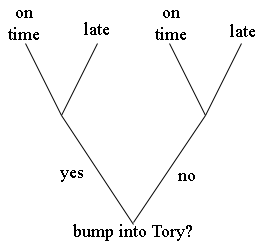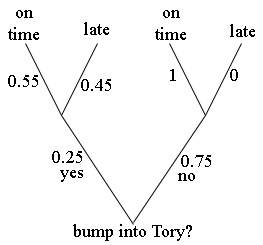SEARCH HOMEMath Central Quandaries & Queriesjuanita bumps into tory in the hallway between period 1 and 2,there is a 45% chance that she will be late for class.if she does not bump into tory, she will make it to class on time. there is a 25% chance that juanita will bump into tory. what is the probability that she will be late on any given day. be sure to introduce your events for this question and show the appropriate formula given the events. --- Tks n bst rgrds AliAli,

I would use a tree diagram to analyze this question. Here is the structure of the tree I have in mind.From the question I can put probabilities on each branch. Juanita bumps into Tory with a probability of 0.25 and hence the probability that she doesn't bump into Tory is 1 - 0.25 = 0.75. If she bumps into Tory then the probability she is late for class is 0.45 and hence the probability that she is on time is 1 - 0.45 = 0.55. Similarly if she doesn't bump into Tory. Thus the tree with probabilities isI hope this helps,
PennyMath Central is supported by the University of Regina and The Pacific Institute for the Mathematical Sciences.